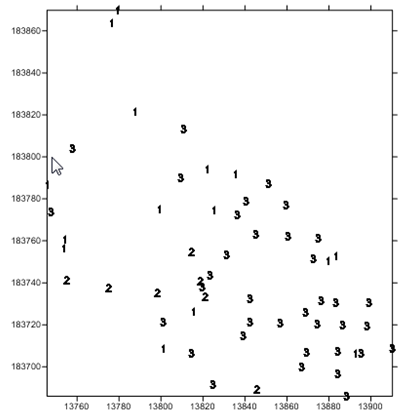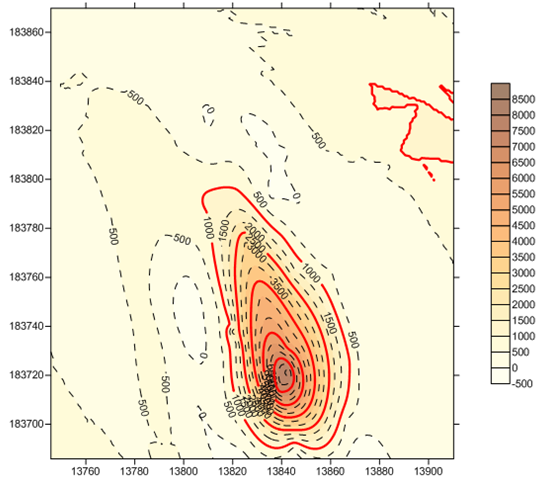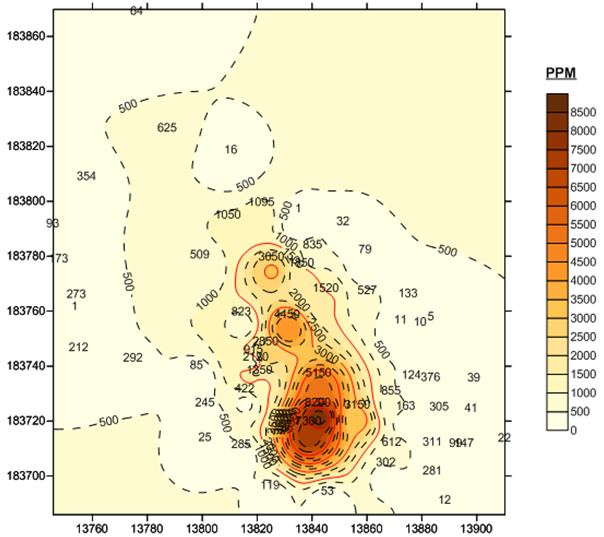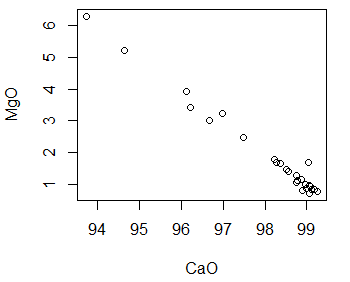# Category Archives: Spatial Statistics

Category for my course in spatial statistics with UNIGIS program at University of Salzburg

# Kriging, IDW, and Other Statistical Exotica

Kriging, Inverse Distance Weighting (IDW) and other statistical algorithms are ways to perform interpolation on a set of data. Where these algorithms are useful is when you have some samples of an area but you want to get an impression of the whole area.Consider, for example, that there has been an oil spill in a certain area, and that engineers have taken samples of the area, measuring for hydrocarbons. They give the samples to you and ask you to provide a picture of the entire area.

You convert the samples to values of 1, 2, or 3, depending on how high the hydrocarbon level is in the sample and put the samples on a map. The samples clearly show the level of hydrocarbons in those sample area. But what about the rest of the area?Here is where interpolation can be useful. You can use these algorithms to estimate values between the samples. Each algorithm provides a different estimate. For example, Kriging shows a gradual progression of values heading toward the lower right of the map.On the other hand, IDW to Power 3 shows a concentration in the lower right of the map.

Which is right? Actually neither is guaranteed to be 100% accurate. Each has its characteristics, its strengths, its weakness. The important point is that interpolation techniques like kriging and Inverse Distance Weighting help us to visualize what areas might look like, given a set of samples of the area. In GIS, being close to accurate is often the best that you can do.

# Spatial Statistics

I’m on the last legs of my program now (not my last legs). My eighth core course to date is Spatial Statistics.

Statistics can help us to understand facts by grouping them into patterns that make sense for the data. For example, consider the graph to the right.It is a scatterplot where each point represents the value of magnesium for a given value of calcium in limestone.

It is clear from the pattern of the points that there is relationship between these two values. As calcium increases, magnesium decreases. But can we define this relationship more precisely?

Looking at the second graph we see a regression line drawn through the points. This line tells us the expected value for magnesium for a given value of calcium.Now there may never be an exact calcium / magnesium value along the regression line. Also, if we add more points to the scatterplot and recalculate, the regression line is likely to change. The point of the regression is not to foretell all future calcium / magnesium points. Rather, it gives us a clear picture of the relationship between calcium and magnesium as it exists now.